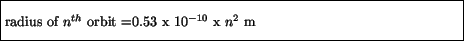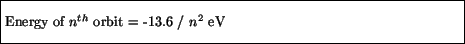Next: Emission spectrum Up: Bohr model Previous: Electrons as de Broglie

## Quantized orbits

Although it appears to be a radical step to consider the electron as a wave just to get a stable orbit, this model of the the atom with an electron wave has some further surprising successes. The condition for a stable orbit is that an integral number of wavelengths of the wave fit around the orbit:where n is any integer. Now, according to de Broglie's hypothesis, the wavelength of the wave is related to the speed of the associated particle. Furthermore, for a particle going around a circle, the speed of the particle is related to the force - remember that a force exists because the direction of motion is constantly changing. This force, which is the electrical force of attraction between the electron and proton, in turn depends on the radius of the orbit. Ultimately, what happens is that the condition for a standing wave pattern is a condition on the radius of the orbit which can be satisfied only by certain values. Numerically, these orbits are given bywhere again n is an integer. The orbitals are thus quantized - they occur in discrete steps. Relating the values of the radii back to an energy through de Broglie's relation, one finds the energy of the electron also is quantized:where 1 eV = 1 electron Volt = 1.6 x 10-19 Joules (the kinetic energy gained by an electron when accelerated through 1 Volt of potential difference). This energy of the electron includes the kinetic energy as well as the electrical potential energy, and by convention is negative - this means that higher energy states have larger values of n.Next: Emission spectrum Up: Bohr model Previous: Electrons as de Broglie
modtech@theory.uwinnipeg.ca
1999-09-29